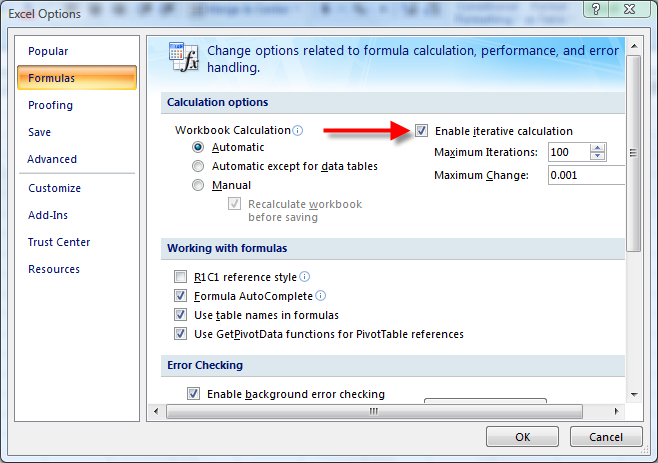Persistent Minimums And Maximums

This page describes how to write formulas for persistent minimums and maximums using Circular References.Introduction

The MIN and MAX functions can return the minimum and maximum values in a range of cells. However, these values will change when any of the values in the test range change. This page describes formulas you can use to return what we will call the persistent minimum and persistent maximum values of a cell or range of cells. The term persistent means that the minimum or maximum value of a cell is kept even if the current minimum or maximum changes. That is, the formula returns the minimum or maximum ever reached by a cell or range. For example, if a cell's value is 10 and then changes to 5, then persistent maximum remains 10 even after the value falls to 5. This is accomplished using Circular References.

A Circular Reference describes the condition in which a formula refers to itself. Most of the time, a circular reference is unintended and for that reason, circular references are by default treated as errors. For example, if you use the formula =A1 in cell A1, this is a circular reference since A1 refers to itself. If you enter this formula, Excel will automatically display the Circular References command bar and the help page for Circular References. You can enable Circular References for the Options dialog, shown below.

Calculation Options in Excel 2003 and earlier.In Excel 2003 and earlier, go to the Tools menu and choose Options. There, choose the Calculation tab and check the Iterations check box, shown above.

Calculation Options in Excel 2007.In Excel 2007, click the Office button (the big circular button in the upper left of the Excel window), click the Excel Options button and then click Formula. There, check the Iterations option.

Persistent Minimum With Reset

The formula below when entered in cell D6 will return the highest value ever reached in cell C6:

```=IF(E6<>"",E6,MIN(D6,C6))
```

This works by calling the MIN function passing to it the value in C6 and the value of the cell D6, the cell containing formula. The formula also uses cell E6 as a reset control. If E6 is empty, the minumum of cells D6 and C6 is returned. If E6 is not empty, the formula returns that value, allowing you to reset the minimum value. Since D6 itself may be less than cell C6, the MIN function will return D6 if it is lower than cell C6. Note that if you edit the formula in D6, its persistence is lost.

You are not limited to testing the minimum against one cell. You can test against the minimum of a range of cells, as shown in the formula below:

```=IF(E15<>"",E15,MIN(C15:C19,D15))
```

When entered in cell D15, this returns the minimum value ever reached in the range C15:C19. It uses cell E15 as the reset cell, allowing you to reset the minimum of a new value not in the range C5:C19 and allowing you to change the values in C15:C19 without affecting the value in D15.

Persistent Maximum With Reset

The formulas for getting the persistent maximum are very similar to the formulas for the persistent minimum.

```=IF(E11<>"",E11,MAX(D11,C11))
```

When entered in cell D11 this formula returns the maximum value ever entered in cell C11. It uses cell E11 as the reset cell. You can return the persistent maximum of a range of cells rather than a single cell with formula below:

```=IF(E23<>"",E23,MAX(D23,C23:C27))
```

When entered in cell D23, this formula returns the maximum value ever reached in the range of cells C23:C27.

Large And Small

You are not limited to the MIN and MAX functions. You can apply the same concepts to the LARGE and SMALL functions. For example,

```=LARGE((C3:C10,D3),2)
```

When entered in cell D3, this will return the persisted second largest value reached in the range C3:C10.

Simlarly, the following formula when entered in D5 will return the second smallest value ever reached in the range C3:C10

```=SMALL((C3:C10,D5),2)
```